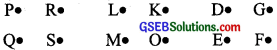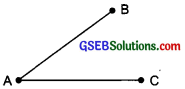# GSEB Solutions Class 6 Maths Chapter 4 Basic Geometrical Ideas InText Questions

Gujarat Board GSEB Textbook Solutions Class 6 Maths Chapter 4 Basic Geometrical Ideas InText Questions and Answers.

## Gujarat Board Textbook Solutions Class 6 Maths Chapter 4 Basic Geometrical Ideas InText Questions

Try These (Page 70)

Question 1.
With a sharp tip of the pencil, mark four points on a paper and name them by the letters A, C, F, H. Try to name these points in different ways. One such way could be thisSolution:
We can name these points in various ways such as:Question 2.
A star in the sky also gives us an idea of a point. Identify at least five such situations in your daily life.
Solution:
Tip of a pencil, tip of a needle, comer of a desk, corner of a paper, tip of a pen.

Try These (Page 71)

Question 3.
Name the line segments in the figure. Is A, the end point of each line segment?Solution:
The line segments are:
(i) $$\overline{\mathrm{AB}}$$ (or $$\overline{\mathrm{BA}}$$)
(ii) $$\overline{\mathrm{AC}}$$ (or $$\overline{\mathrm{CA}}$$)
‘Yes, A’ is the end point of each line segment.Try These (Page 74)

Question 1.
1. Name the rays given in this picture.
2. Is T a starting point of each of these rays?Solution:
1. Rays in figure are: $$\overline{\mathrm{TA}}$$, $$\overline{\mathrm{TN}}$$, $$\overline{\mathrm{TB}}$$ and $$\overline{\mathrm{NB}}$$
2. No. [ T is not the starting point of $$\overline{\mathrm{NB}}$$]